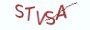# 1.2.1 算法思路# 1.2.2 算法实现

```# __author__ = 薯条老师
import random
x = random.randint(0, 1000)
y = random.randint(0, 1000)
z = random.randint(0, 1000)

# 假定最小值为x
min_ = x

# 如果y比min_还要小，那么将y指向的值赋值给min_
if y < min_:
min_ = y
# 如果z比min_还要小，那么将z指向的值赋值给min_
elif z < min_:
min_ = z

print("min:{}".format(max_))

"""
# 更简洁的代码
min_ = x if x < y else y
if z < min_:
min_ = z
"""```

# 1.2.3 最具实力的小班培训

`(1) Python后端工程师高薪就业班，月薪11K-18K，免费领取课程大纲(2) Python爬虫工程师高薪就业班，年薪十五万，免费领取课程大纲(3) Java后端开发工程师高薪就业班，月薪11K-20K, 免费领取课程大纲(4) Python大数据分析，量化投资就业班，月薪12K-25K,免费领取课程大纲`## 注册账号